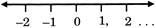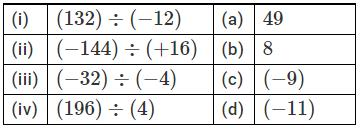Courses

# Test: Integers - 3

## 20 Questions MCQ Test Mathematics (Maths) Class 7 | Test: Integers - 3

Description
This mock test of Test: Integers - 3 for Class 7 helps you for every Class 7 entrance exam. This contains 20 Multiple Choice Questions for Class 7 Test: Integers - 3 (mcq) to study with solutions a complete question bank. The solved questions answers in this Test: Integers - 3 quiz give you a good mix of easy questions and tough questions. Class 7 students definitely take this Test: Integers - 3 exercise for a better result in the exam. You can find other Test: Integers - 3 extra questions, long questions & short questions for Class 7 on EduRev as well by searching above.
QUESTION: 1

### What do we call the set of negative numbers and whole numbers?

Solution:

The set of negative numbers and whole numbers is called integers.

Z={,....−2,−1,,0,1,2,.....}

QUESTION: 2

### Which of the following is the smallest positive integer?

Solution:

1 is the smallest positive integer.

QUESTION: 3

### Where are the negative numbers located on a horizontal number line?

Solution:

All the other positive numbers to its right are greater than, the negative numbers are located on the left side of 0.QUESTION: 4

What is the opposite of earning Rs. 100?

Solution:

The required opposite is spending Rs. 100.

QUESTION: 5

How is the withdrawal of RS. 200 represented?

Solution:

Withdrawal of Rs. 200 means amount 200 is subtracted from our account. Option B is correct.

QUESTION: 6

Which of the following is true with respect to −28 and −32?

Solution:

Because -32 is on the left of -28 on the number line, so it is smaller.

QUESTION: 7

Where do we place the positive numbers on a vertical number line with respect to 0?

Solution:

On a vertical number linepositive numbers are located above zero.

QUESTION: 8

What is the representation of 30 km towards the west?

Solution:

Since west is on the left side, and there are negative integers on the left, so it's -30 kms.

QUESTION: 9

What is the nature of the product of a negative integer by itself, odd number of times?

Solution:

(−1)×(−1)×(−1)........odd times

= (−1)  which is negative.

QUESTION: 10

What is the nature of the product of a negative number by itself even number of times?

Solution:

(−1)×(−1)×(−1)....even times =1,which is positive.

QUESTION: 11

A Calculate (−32)×(−4)×(−3)×0×(−6)

Solution:

(−32)×(−4)×(−3)×0×(−6)=0 as the product of any number and 0 is 0.

QUESTION: 12

If the dividend and the divisor have like signs, what is the sign of the quotient?

Solution:

If dividend and divisor have positive signs then the quotient will be positive. If dividend and divisor have negative signs , then the quotient will be positive because 2 negative signs becomes positive.

QUESTION: 13

If the dividend and divisor have unlike signs, what is the sign of the quotient?

Solution:
QUESTION: 14

Match the following.Solution:

(132) / (-12) = -11

(-144) / (+16) = -9

(-32) / (-4) = 8

(196) / (4) = 49

QUESTION: 15

With respect to which of the following operations is closure property satisfied by the set of integers?

Solution:

The closure property states that when you perform an operation on any two numbers in a set, the result of the computation is another number in the same set .Since division can result in changing an integer in decimals. So its closed in addition, subtraction and multplication.

QUESTION: 16

What is the additive identity element for the set of integers?

Solution:

0 is identity element with respect to addition of integers, e.g.,(−5)+0 = +0+(−5) = (−5)

QUESTION: 17

Which of the following is the multiplicative identity in the set of integers?

Solution:

1 is the identity element with respect to multiplication.

QUESTION: 18

What is the value of 124×4−3+118÷2?

Solution:

Use BODMAS rule & simplify:

= 124×4  −3 + 118+2

= 496 − 3 + 59

= 552

QUESTION: 19

Which of the following order is used while evaluating an expression?

Solution:

(),{},[] is the correct order of evaluation of brackets.

QUESTION: 20

If a negative sign precedes a bracket, what happens to the terms inside it?

Solution:

If a negative sign precedes a bracket, the signs of the terms inside the bracket are changed.